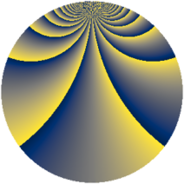# Properties

 Label 1104.2.uLevel $1104$ Weight $2$ Character orbit 1104.u Rep. character $\chi_{1104}(323,\cdot)$ Character field $\Q(\zeta_{4})$ Dimension $352$ Sturm bound $384$

# Related objects

## Defining parameters

 Level: $$N$$ $$=$$ $$1104 = 2^{4} \cdot 3 \cdot 23$$ Weight: $$k$$ $$=$$ $$2$$ Character orbit: $$[\chi]$$ $$=$$ 1104.u (of order $$4$$ and degree $$2$$) Character conductor: $$\operatorname{cond}(\chi)$$ $$=$$ $$48$$ Character field: $$\Q(i)$$ Sturm bound: $$384$$

## Dimensions

The following table gives the dimensions of various subspaces of $$M_{2}(1104, [\chi])$$.

Total New Old
Modular forms 392 352 40
Cusp forms 376 352 24
Eisenstein series 16 0 16

## Trace form

 $$352q + 6q^{6} + O(q^{10})$$ $$352q + 6q^{6} + 6q^{12} - 20q^{18} - 40q^{22} - 52q^{24} - 12q^{27} - 24q^{28} - 68q^{30} - 34q^{36} - 24q^{39} - 20q^{42} - 32q^{43} + 352q^{49} - 40q^{51} - 8q^{52} + 100q^{54} - 64q^{55} - 36q^{58} + 44q^{60} - 84q^{64} - 92q^{66} - 144q^{70} - 60q^{72} + 56q^{75} - 96q^{76} - 70q^{78} + 4q^{82} - 64q^{84} + 112q^{87} + 152q^{88} + 12q^{90} + 48q^{91} - 24q^{93} - 24q^{94} + 40q^{96} + 64q^{99} + O(q^{100})$$

## Decomposition of $$S_{2}^{\mathrm{new}}(1104, [\chi])$$ into newform subspaces

The newforms in this space have not yet been added to the LMFDB.

## Decomposition of $$S_{2}^{\mathrm{old}}(1104, [\chi])$$ into lower level spaces

$$S_{2}^{\mathrm{old}}(1104, [\chi]) \cong$$ $$S_{2}^{\mathrm{new}}(48, [\chi])$$$$^{\oplus 2}$$